Courses

# NCERT Solutions(Part - 2) - Practical Geometry Class 7 Notes | EduRev

## Class 7 : NCERT Solutions(Part - 2) - Practical Geometry Class 7 Notes | EduRev

The document NCERT Solutions(Part - 2) - Practical Geometry Class 7 Notes | EduRev is a part of the Class 7 Course Mathematics (Maths) Class 7.
All you need of Class 7 at this link: Class 7

Exercise 10.4

Question 1:

Construct ΔABC, given m∠A = 60:, m∠B = 30° and AB = 5,8 cm.

To construct: ΔABC, given m∠A = 60:, m∠B = 30° and AB = 5,8 cm.

Steps of construction:

(a) Draw a line segment AB = 5.8 cm.
(b) At point A, draw an angle Z YAB = 6ti= with the help of a compass.
(c) At point B, draw Z XBA = 30" with the help of a compass.
(d) AY and BX intersect at the point C.
It is the required triangle ABC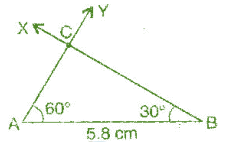Question 2:

Construct Δ PQR if PQ = 5 cm, m∠PQR = 105° and m∠.QRP = 40°.

Given:

m∠ PQR = 105° and m∠ QRP = 40°
We know that sum of angles of a triangle is 180°.
∴ m∠PQR + m∠QRP + m∠ QPR = 180°
⇒ 105° + 40° + m∠QPR= 180°
⇒ 145° + m∠QPR= 180°
⇒  m∠QPR= 180° - 145°
⇒ m∠QPR = 35°

To construct: ΔPQR where m∠P = 35°, m∠Q = 105° and PQ = 5 cm.

Steps of construction:

(a) Draw a line segment PQ = 5 cm,
(b) At point P, draw ∠ XPQ = 35° with the help of protractor.
(c) At point Q, draw ∠ YQP = 105° with the help of protractor.
(d) XP and YQ intersect at point R.
It is the required triangle PQR.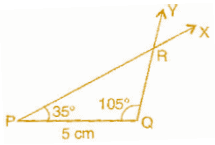Question 3:

Examine whether you can construct ΔDEF such that EF = 7.2 cm, m∠ E= 110° and m∠F

Given: In ΔDEF, m∠E = 110° and m∠T = 80°
Using angle sum property of triangle
∠D + ∠E + ∠F = 180°
⇒∠D + 110°+ 80° = 180°
⇒ ∠D + 190° = 180°
⇒ ∠D = 180° - 190° = -10°

Which is not possible.

Exercise 10.5

Question 1:

A right angled triangle PQR where m∠Q = 9ff, QR= 8 cm and PQ = 10 cm.

To construct: Construct the right angled Δ PQR, where m∠Q = 90", QR = 8 cm and PR = 10 cm.

Steps of construction: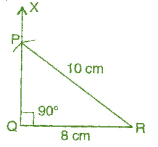(a)  Draw a line segment QR = 8 cm,
(b) At point Q, draw QX ⊥ QR,
(c) Taking R as centre, draw an arc of radius 10 cm.
(d) This arc cuts QX at point P.
(e) foin PQ.
It is the required right-angled triangle PQR,

Question 2:

Construct a right-angled triangle whose hypotenuse is 6 cm long and one the legs is 4 cm long.

To construct: A right-angled triangle DEF where DF = 6 cm and EF = 4 cm

Steps of construction:

(a) Draw a line segment EF = 4 cm.
(b) At point Q, draw EX ⊥ EF.
(c) Taking F as centre and radius 6 cm, draw an arc. (Hypotenuse)
(d) This arc cuts the EX at point D.
(e) Join DF.
It is the required right-angled triangle DEF.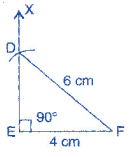Question 3:

Construct an isosceles right angled triangle ABC, where m∠ACB = 90° and AC = 6 cm.

To construct:  An isosceles right angled triangle ABC where m∠ C = 90% AC = BC = 6 cm.

Steps of construction:

(a) Draw a line segment AC = 6 cm.
(b) At point C, draw XC ⊥ CA.
(c) Taking C as centre and radius 6 cm, draw an arc.
(d) This arc cuts CX at point B.
(e) Join BA.
It is the required isosceles right-angled triangle ABC.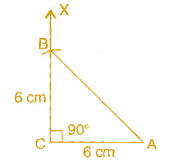Miscellaneous Questions

Questions:

Below are given the measures of certain sides and angles of triangles. Identify those which cannot be constructed and say why you cannot construct them. Construct rest of the triangle.

 Triangle Given measurements 1. ΔABC m∠A = 85° ; m∠B = 115° ; AB = 5 cm 2. ΔPQR m∠ Q = 30° ; m∠R = 60° ; QR = 4.7 cm 3. ΔABC m∠ A = 70° ; m∠B = 50°; AC = 3 cm 4. ΔLMN m∠L = 60° ; m∠N = 120° ; LM = 5 cm 5. ΔABC BC = 2 cm; AB = 4 cm; AC = 2 cm 6. ΔPQR PQ = 3.5 cm; QR = 4 cm; PR = 3.5 cm 7. ΔXYZ XY = 3 cm; YZ = 4 cm; XZ = 5 cm 3. ΔDEF DE = 4.5 cm; EF = 5.5 cm; DF = 4 cm

In ΔABC, m∠A = 85o , m∠B = 115o , AB = 5 cm

Construction of ΔABC is not possible because m∠A = 85o + m∠B = 200o, and we know that the sum of angles of a triangle should be 180o

To construct: ΔPQR where m∠Q = 30o , m∠R = 60o and QR = 4.7 cm.

Steps of construction:

(a) Draw a line segment QR = 4.7 cm.
(b) At point Q, draw ∠XQR = 30' with the help of a compass.
(c) At point R, draw ∠YRQ = 60' with the help of a compass.
(d) QX and RY intersect at point P.
It is the required triangle PQR.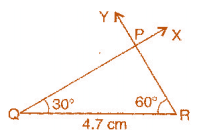We know that the sum of angles of a triangle is 180°.
m∠ A + m∠B + m∠C = 180°
∴ 70° + 50° + m∠C = 180°
⇒  120° + m∠C = 180°
⇒  m∠C = 180° - 120°
⇒  m∠C = 60°

To construct: ΔABC where m∠A = 70°, m∠C = 60° and AC = 3 cm.

Steps of construction:

(a) Draw a line segment AC = 3 cm.
(b) At point C, draw ∠YCA = 60°.
(c) At point A, draw ∠XAC = 70°
(d) Rays XA and YC intersect at point B

It is the required triangle ABC.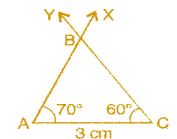In ΔLMN , m∠L - 60° m∠N = 120°, LM = 5 cm
This ΔLMN is not possible to construct because m∠L + m∠N = 60° + 120° = 180° which forms a linear pair.

Δ ABC, BC = 2 cm, AB = 4 cm and AC = 2 cm

This Δ ABC is not possible to construct because the condition is

Sum of lengths of two sides of a triangle should be greater than the third side.

AB< BC + AC
⇒ 4 < 2 + 2
⇒ 4 = 4,

To construct: ΔPQR where PQ = 3.5 cm, QR = 4 cm and PR = 3.5 cm

Steps of construction:

(a) Draw a line segment QR = 4 cm.
(b) Taking Q as centre and radius 3.5 cm, draw an arc.
(c) Similarly, taking R as centre and radius 3.5 cm, draw another arc which intersects the first arc at point P.
it is the required triangle QPR.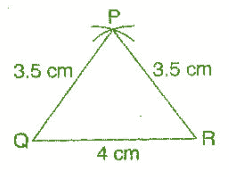To construct: A triangle whose sides are XY = 3 cm, YZ = 4 cm and XZ = 5 cm.

Steps of construction:

(a) Draw a line segment ZY = 4 cm.
(b) Taking Z as center and radius 5 cm, draw an arc.
(c) Taking Y as centre and radius 3 cm, draw another arc.
(d) Both arcs intersect at point X.
It is the required triangle XYZ.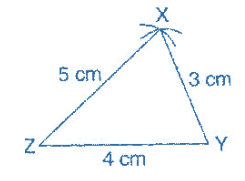To construct: A triangle DEF whose sides are DE = 4.5 cm, EF = 5.5 cm and DF = 4 cm.

Steps of construction:

(a) Draw a line segment EF = 5.5 cm.
(b) Taking E as center and radius 4.5 cm, draw an arc.
(c) Taking F as center and radius 4 cm, draw another arc which intersects the first arc at point D.

It is the required triangle DEF.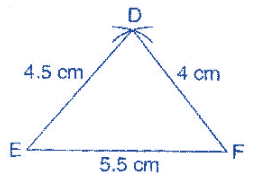Offer running on EduRev: Apply code STAYHOME200 to get INR 200 off on our premium plan EduRev Infinity!

## Mathematics (Maths) Class 7

161 videos|237 docs|45 tests

,

,

,

,

,

,

,

,

,

,

,

,

,

,

,

,

,

,

,

,

,

;